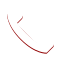Machine Learning & Deep Learning (AI) 2020-04-15T06:29:38+00:00

# Machine Learning & Deep Learning (AI)

Get familiar with Machine Learning & Deep Learning (AI).

INR 60,000 + Taxes

# Machine Learning & Deep Learning (AI)

Machine Learning is the arena of study that bounces computers and it’s a capability to learn without being exclusively programmed. Machine Learning is one of the most sensational technologies that one would have ever come across. As it is unmistakable from the name, it gives the computer that which makes it more similar to humans: The ability to learn, Machine learning is actively being used across the global businesses & society for several reasons & solutions.

Arthur Samuel, an American pioneer in the field of computer gaming and artificial intelligence, coined the term “Machine Learning” in 1959 while at IBM. John McCarthy, an American computer scientist pioneer and inventor, was known as the father of Artificial Intelligence (AI) after playing a seminal role in defining the field devoted to the development of intelligent machines using Machine Learning Techniques.

It is also considered as a subset of artificial intelligence. Machine learning algorithms construct a mathematical model centered on sample data,  generally that is termed as “training data”, in demand to make predictions or decisions without being unambiguously programmed to perform the certain tasks & activities. Machine learning algorithms are used in a wide variety of applications, such as email filtering and computer vision and several others, where it is problematic or to develop a conventional algorithm for effectively performing the task.

At Integrum Litera we begin with training in detail about Introduction to Machine Learning , overview of Machine Learning, applications of Machine Learning, skills required for Machine Learning, Algorithms involved in Machine Learning, Introduction to Deep Learning, basic machine learning algorithms, advanced machine learning algorithms, time series analysis and forecasting, deep learning, neural networks, Image & text processing & lab sessions led by professionals, hands on real time projects, exclusive placement assistance followed by mock interviews and certification.

## CURRICULUM

#### Module 01: Statistical Learning

Module 1: Statistical Learning:

• What Is Statistical Learning?
• Why Estimate f?
• How Do We Estimate f?

1. The Trade-Off Between Prediction Accuracy and Model Interpretability

• Supervised Versus Unsupervised Learning
• Regression Versus Classification Problems
• Assessing Model Accuracy
• Measuring the Quality of Fit

• 2. Linear Regression:

Simple Linear Regression:
• Estimating the Coefficients
• Assessing the Accuracy of the Coefficient Estimates
• Assessing the Accuracy of the Model

3. Multiple Linear Regression:

• Estimating the Regression Coefficients
• Some Important Questions
• Other Considerations in the Regression Model
• Qualitative Predictors
• Interaction Terms
• Non-linear Transformations of the Predictors
• Extensions of the Linear Model
• Potential Problems

4. Classification:
• An Overview of Classification
• Why Not Linear Regression

5. Logistic Regression:
• The Logistic Model
• Estimating the Regression Coefficients
• Making Predictions
• Multiple Logistic Regression
• Logistic Regression for >2 Response Classes

6. Resampling Methods:
• Cross-Validation:
• The Validation Set Approach
• Leave-One-Out Cross-Validation
• k-Fold Cross-Validation
• Cross-Validation
• Cross-Validation on Classification Problems
• The Bootstrap

7. Linear Model Selection and Regularization:
• Subset Selection
• Best Subset
• election Stepwise
• Selection Forward and Backward Stepwise Selection
• Choosing the Optimal Model

8. Shrinkage Methods:
• Ridge Regression
• The Lasso Regression K-Nearest Neighbor

#### Module 02: Deep dive into Machine Learning

Module 2 : Deep dive into Machine Learning:

Tree-Based Methods

1. Basics of Decision Trees:

• Regression Trees
• Classification Trees
• Trees Versus Linear Models

2. Bagging, Random Forests, Boosting:

• Bagging
• Random Forests
• Boosting

3. Support Vector Machines:

• Hyperplane
• The Maximal Margin Classifier
• Support Vector Classifiers
• Support Vector Machines
• Kernel Trick
• Gamma, Cost and Epsilon
• SVMs with More than Two Classes

#### Module 03: Unsupervised Learning

Module 3: Unsupervised Learning:

• The Challenges of Unsupervised Learning
• Principal Components Analysis:
• What Are Principal Components?
• Another Interpretation of Principal Components
• More on PCA
• Other Uses for Principal Components

Clustering Methods:

• K-Means Clustering
• Hierarchical Clustering
• Practical Issues in Clustering

#### Module 04: Association Rules Mining and Times Series Analysis

Module 4: Association Rules Mining and Times Series Analysis

1. Association Rules Mining:

• Apriori/Support/Confidence/Lift

2. Time Series Analysis:

• What is Times Series Data?
• Stationarity in Time Series Data
• Augmented Dickey Fuller Test
• The Box-Jenkins Approach
• The AR Process
• The MA Process
• What is ARIMA?
• ACF,
• PACF and IACF plots
• Decomposition of Times Series
• Trend, Seasonality and Cyclic
• Exponential Smoothing
• EWMA

#### Module 05: Neural Networks, Deep Learning & Practical Issues

Module 5: Neural Networks, Deep Learning & Practical Issues:

Introduction to Neural Networks and Deep Learning:

• Units/Neurons
• Weights/Parameters/Connections
• Single Layer Perceptron
• Multilayer Perceptron

Activation Functions:
• Sigmoid
• Tanh
• ReLU
• Leaky ReLU
• Convolutional Neural Networks
• Recurrent Neural Networks
• Image Processing
• Natural Language Processing

#### What is Machine Learning?

Machine learning is an application of artificial intelligence (AI) that gives frameworks the ability to consequently learn and improve from experience without being expressly programmed.

#### What are the Types of Machine Learning?

Machine learning is sub-categorized to three types:

1. Supervised Learning
2. Unsupervised Learning
3. Reinforcement Learning

#### What is a Random Forest?

A Random Forest is a supervised machine learning algorithm that is commonly used for classification problems.Call Now